Shweta Rawat — Published On January 15, 2023 and Last Modified On January 15th, 2023

## Introduction

A bot text corpus is a collection of texts generated by a bot, a software program designed to perform automated tasks, such as responding to user input or scraping data from the internet.

On the other hand, a human text corpus is a collection of texts humans have written. Human texts may be written in various styles and formats, including narrative, argumentative, descriptive, etc.

The main difference between bot texts and human texts is that a machine generates the bot text, while humans write the latter.

Our DataHour speaker, Sumeet, will give you a practical walkthrough on a collection of Human Text Corpus for bilinguals (English and Hindi) and apply pre-processing techniques to clean it.

About Expert: Sumeet Lalla, Data Scientist at Cognizant, has completed his Masters in Data Science from the Higher School of Economics Moscow and Bachelor of Engineering in Computer Engineering from Thapar University. With 5.5 years of experience in Data Science and Software Engineering, he currently works as a Data Scientist at Cognizant.

## Distinguishing Bot Text from Human Text Corpus

Firstly, we need to collect and pre-process the English/Hindi text. We are using the Gutenberg API to collect English literature novel indices. We are using a jupyter notebook. Side by side, using an ITK and Spacey to do our pre-processing. Now, install tokenizers like “stopwords” and “wordnet” for lemmatization. We have initialized all of these. As you can see below, there is a gutendex web API from which we can pass the relevant parameters.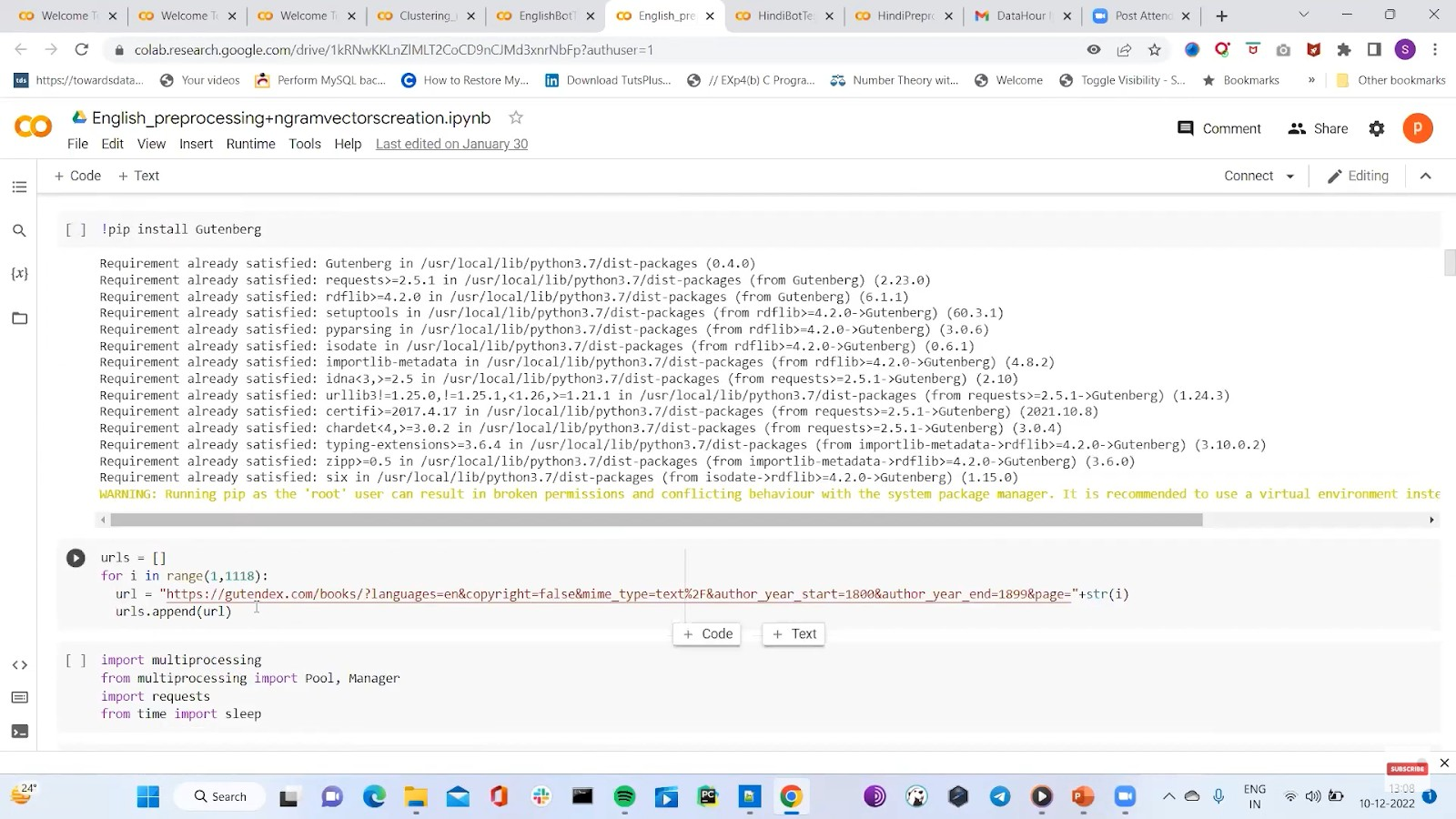We would use ‘gutenberg_cleaner’ in the python library to clean irrelevant headers and volumes. Because we only require the text, chapter names, and the title of the book here. Collect all this information in a separate folder. Now for pre-processing, we will remove all the phrases which are not required, like won’t, can’t, etc., will not, and cannot, respectively. It is needed to clean the text. Also, capitalize the first letter of the sentence. We are using NLP for this.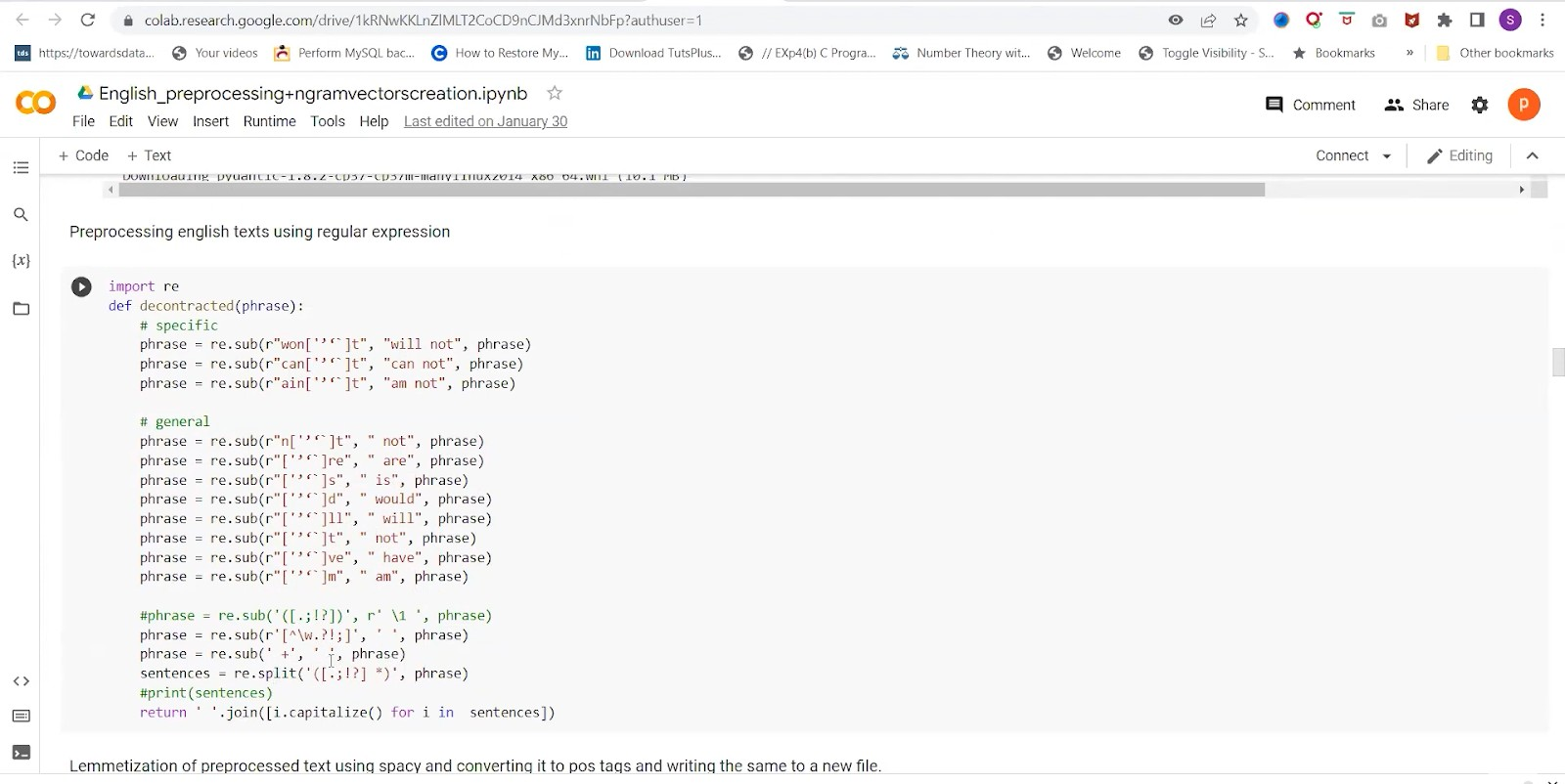We need to create a post dictionary so that the person’s name can be substituted with real names. This is part of speech tagging. As you can see below, here we are initializing the multiprocessing. We are using the pool and map to do it. We are getting the CPU count using “multiprocessing.cpu_count”. This will run the pre-processing function which we discussed earlier.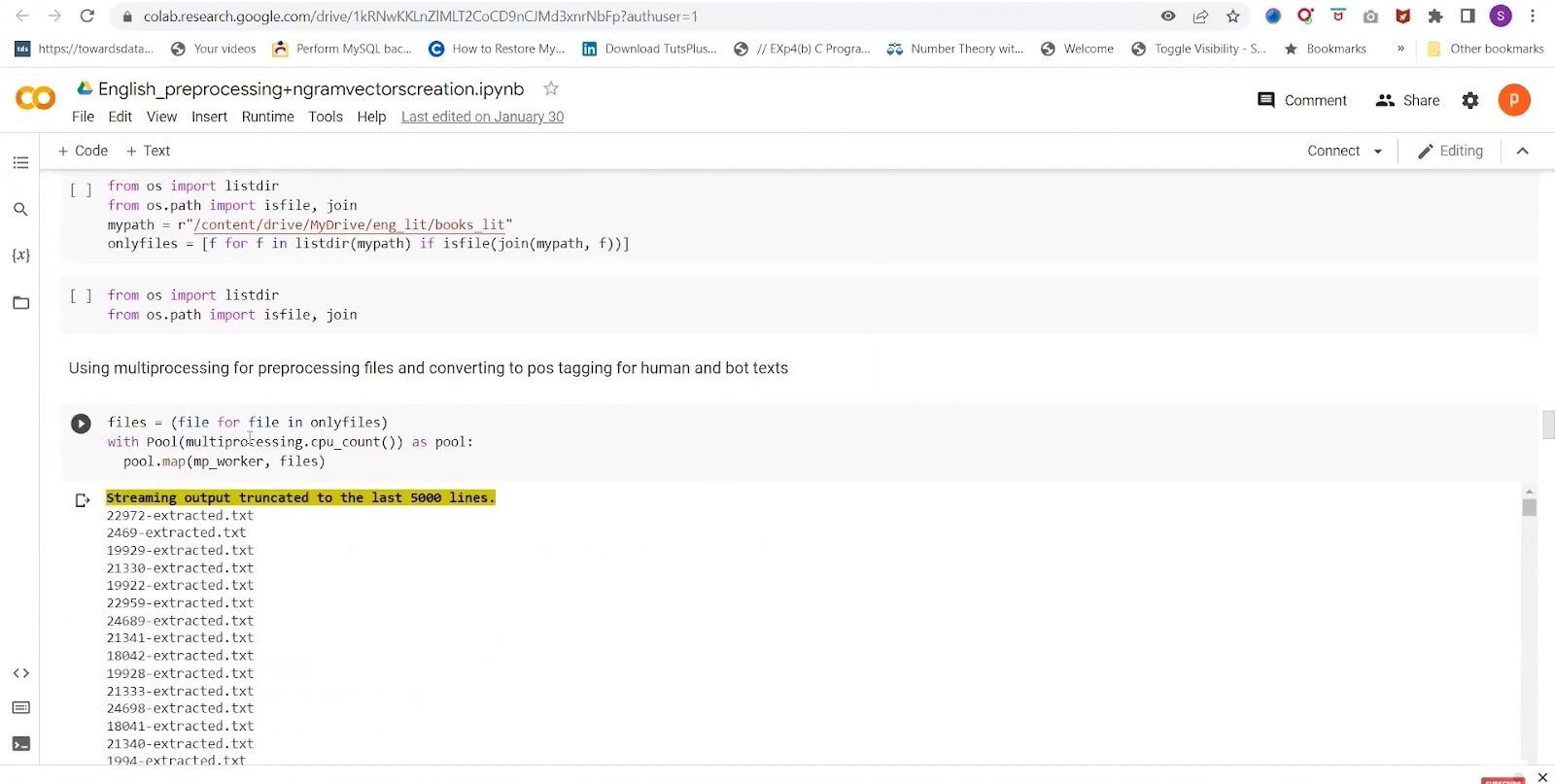We are creating a corpus for this file. We would append all the previously cleaned elements we had done into the single output file, which is named “english_corpus.txt.”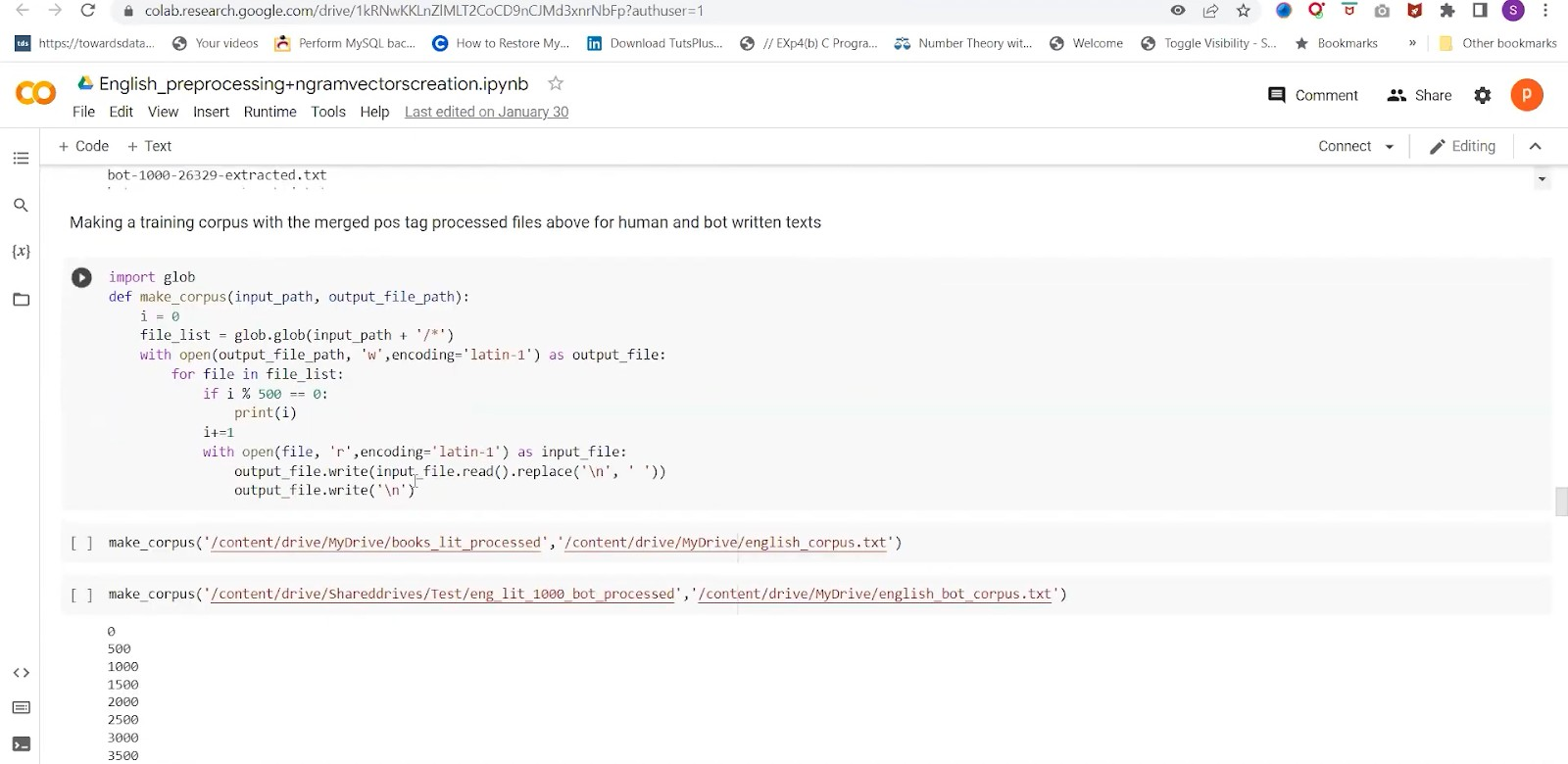Now we are using John Snow spark NLP as it has a pre-trained pipeline for lemmatization and tokenization. Using pyspark here and setting up the document assembler for setting up the tokenizer. We are using a Hindi pretend symmetrized and will get the Hindi text as required. We need to do some manual work here.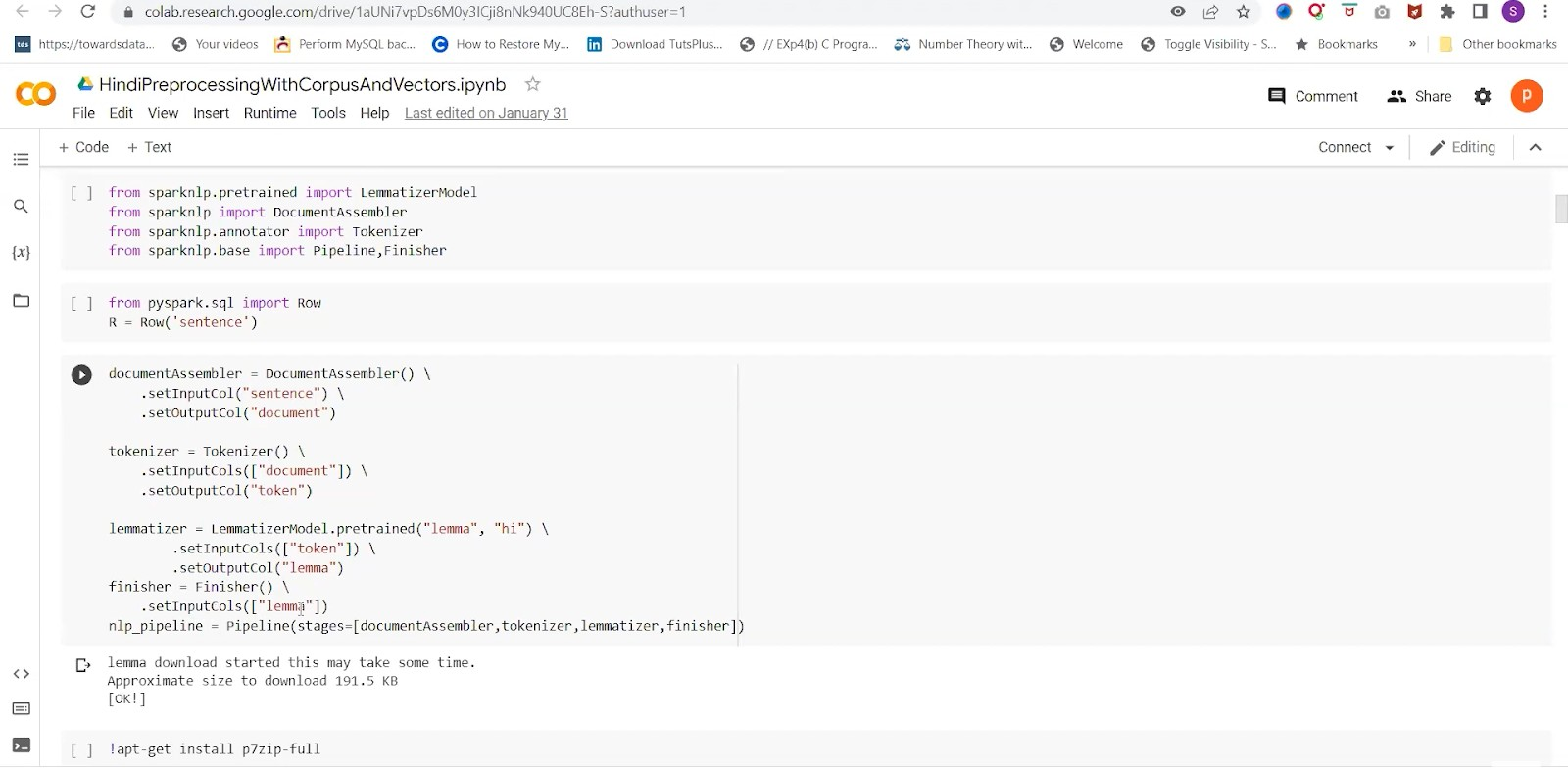Here, we have created “get_lemmatized_file” for the pre-processing. Same as the English text, here we are creating an NLP spark pipeline and would select the final column as the finished lemma. This would be the final process text. This should be done for all the hindi files.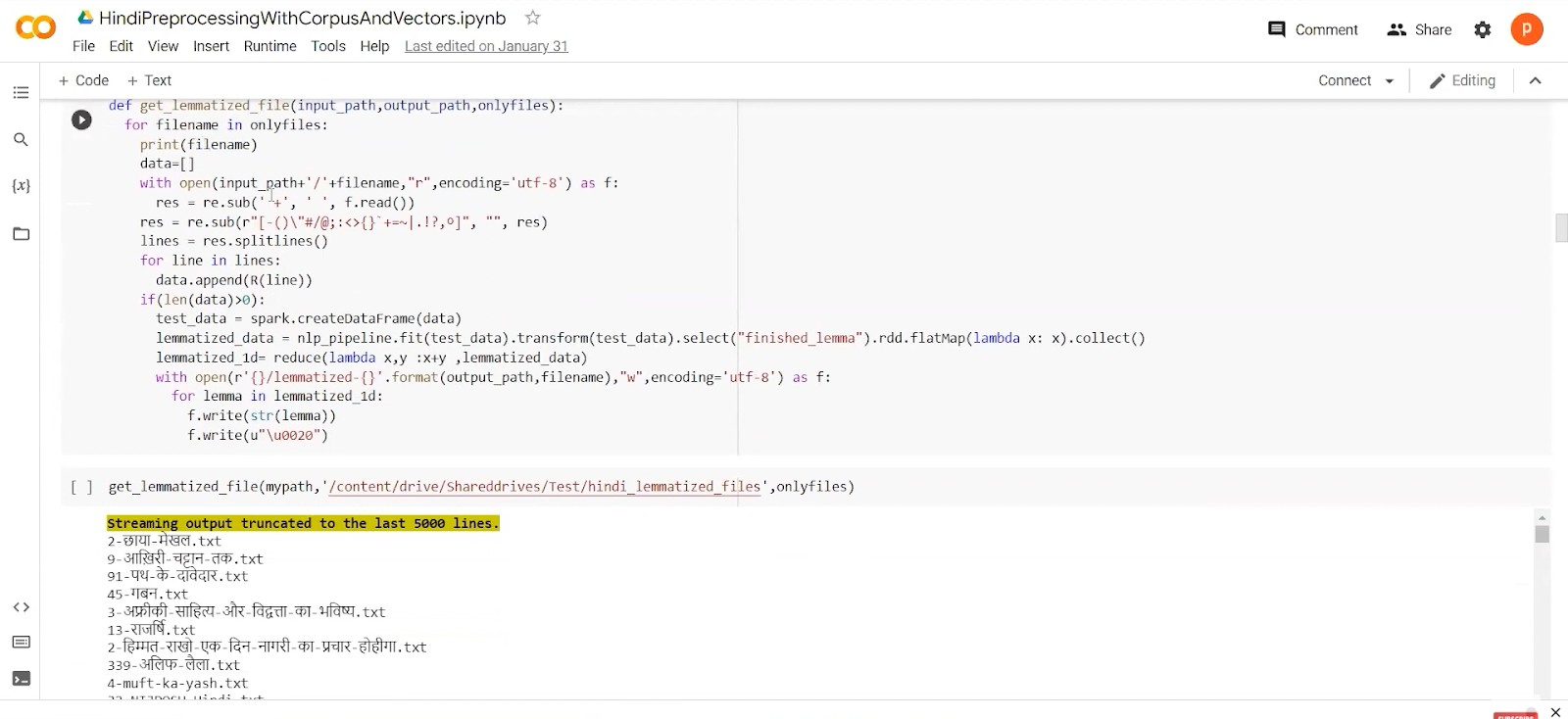Now going back to English again, We need to apply TF-IDF and SVD to generate word vectors. First, we would perform TF Vectorisation of pre-processed text. For that, you need to put an analyzer as a word. SVD is used to reduce the dimensionality of the TF-IDF matrix. Now we have to choose a low-rank k approximation using the Eckart-Young theorem.

The below slide explains the SVD used for low-rank k approximation to get word vectors.

### Basic definitions of SVD.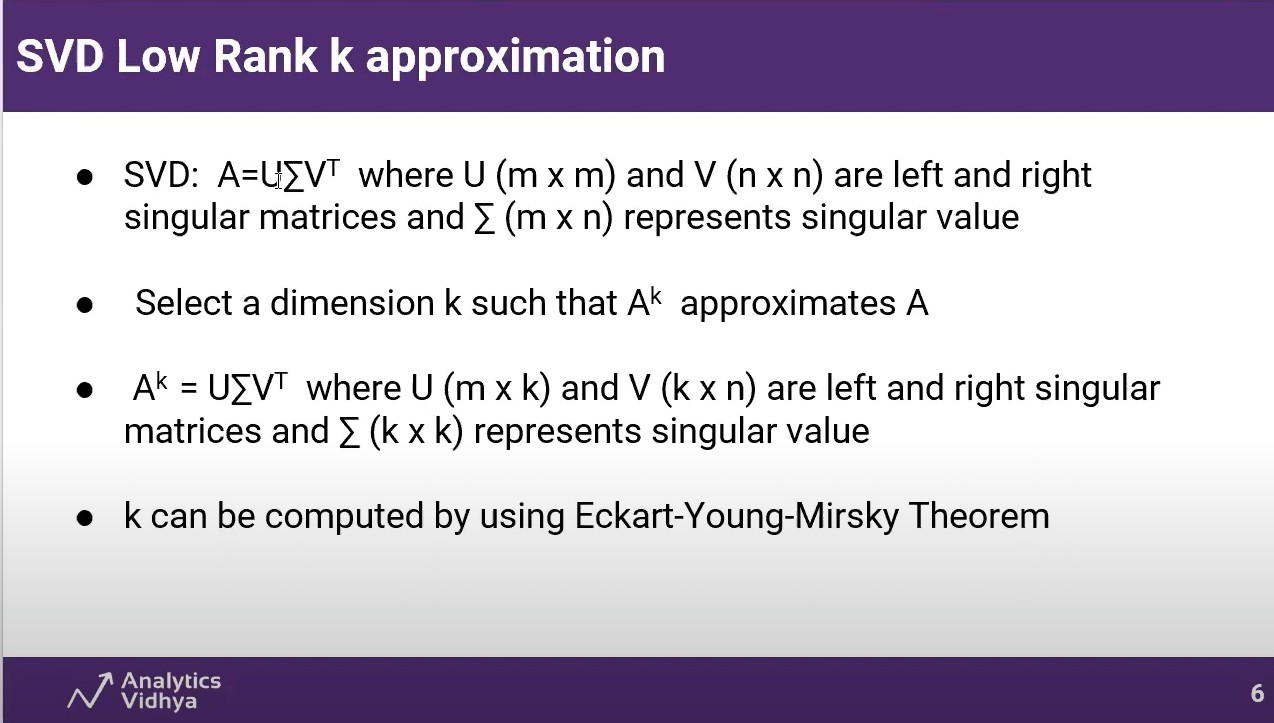We can find the value of A using the Eckart-Young-Mirsky Theorem.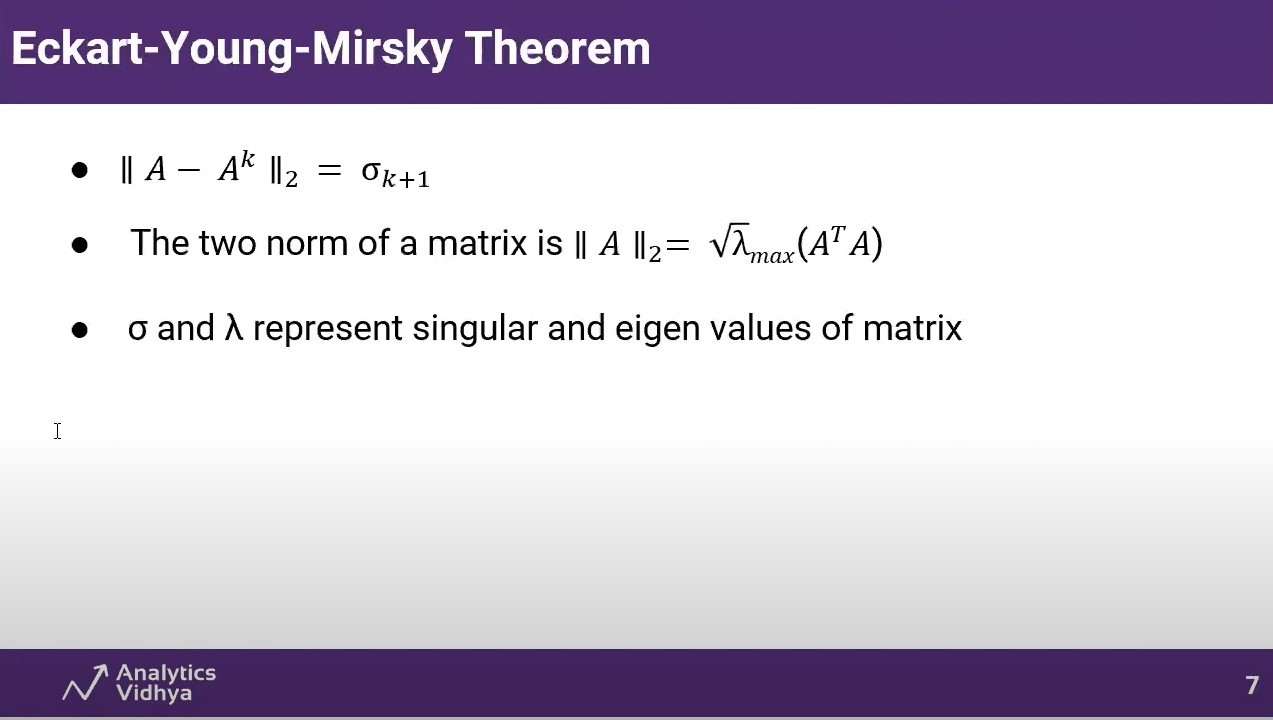For our approach, it came out to be 10. We are decomposing the English vectors into u, sigma and vt matrix. You will get the row-subspace of the matrix.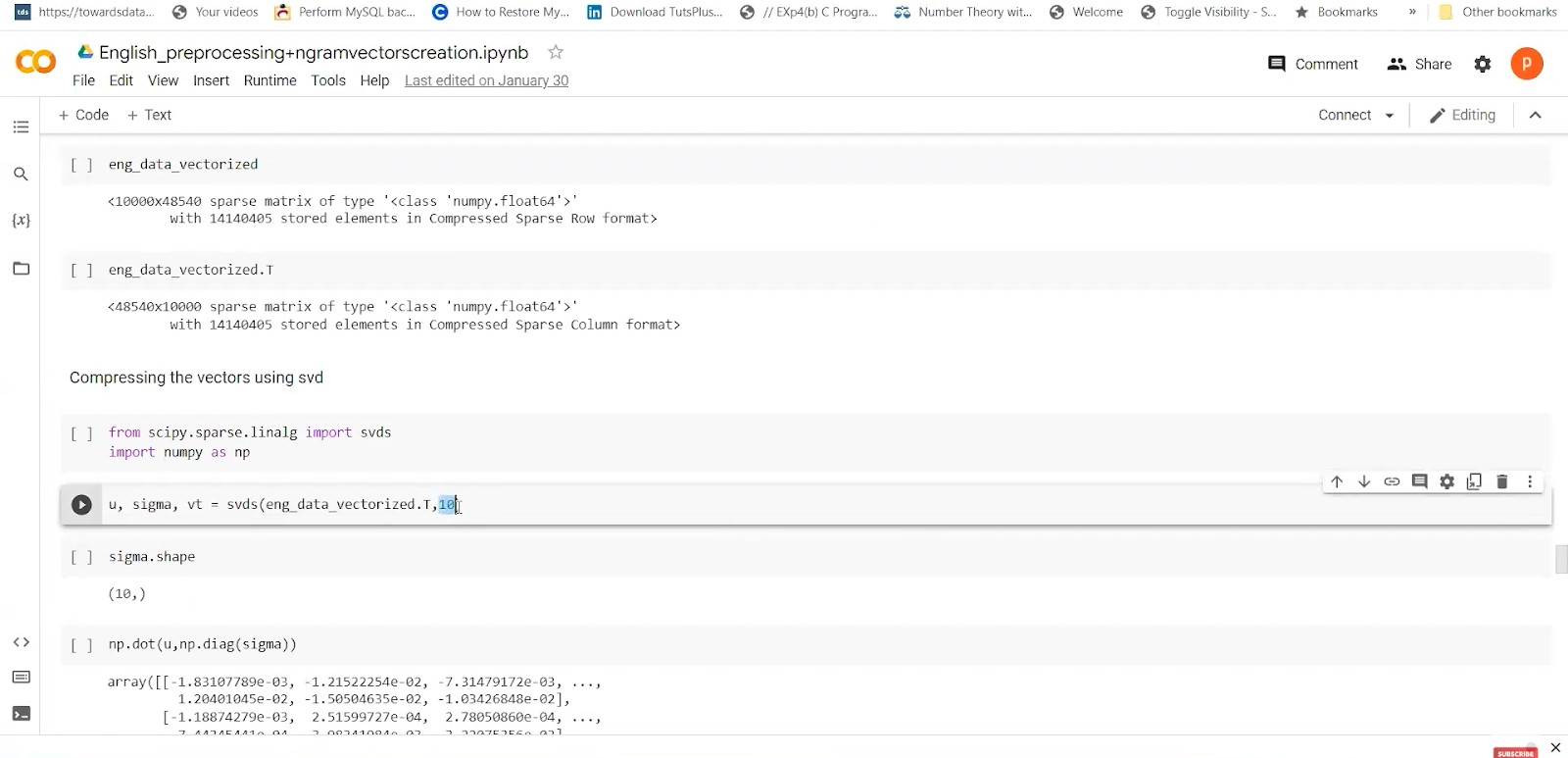#### Word vector space from reduced SVD Matrix:For our data, we have u-sigma as row-subspace to represent the whole word vectors in the space. We are using binary search to get the English vectors. Those vectors will be stored in the dictionary for faster use. We need to do stripping for pre-processing and then append it to the file and dictionary. So basically, we will search for the word in the dictionary and get the corresponding vectors. As you can see in the screenshot below, the ‘Speak’ word is represented as a dimension with a column dimension of 10.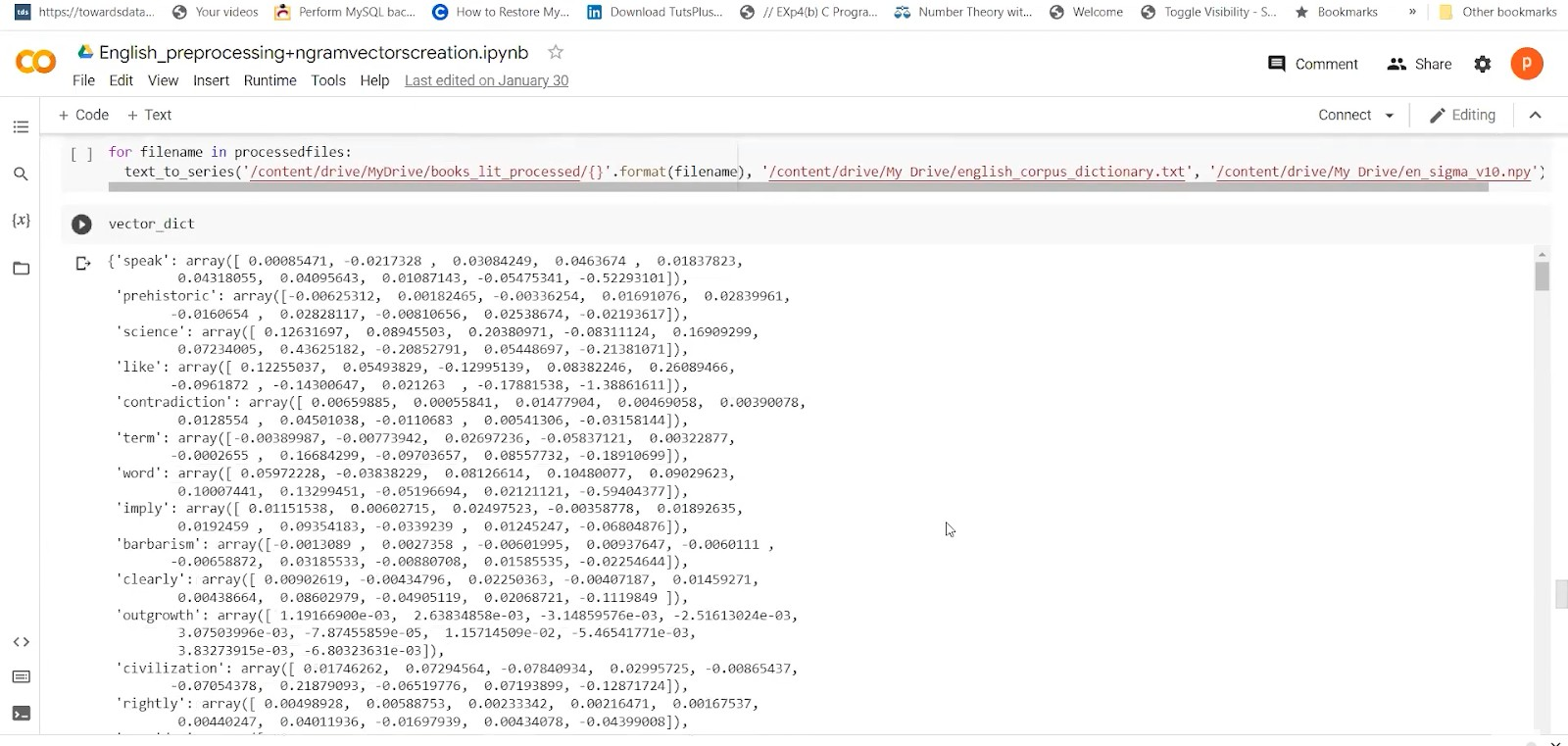Now, we are moving to the next step, which is generating the n-grams. This is the simplest way of getting the word vectors. To generate n-gram vectors, we have helper functions. Need to give range to the n-grams function.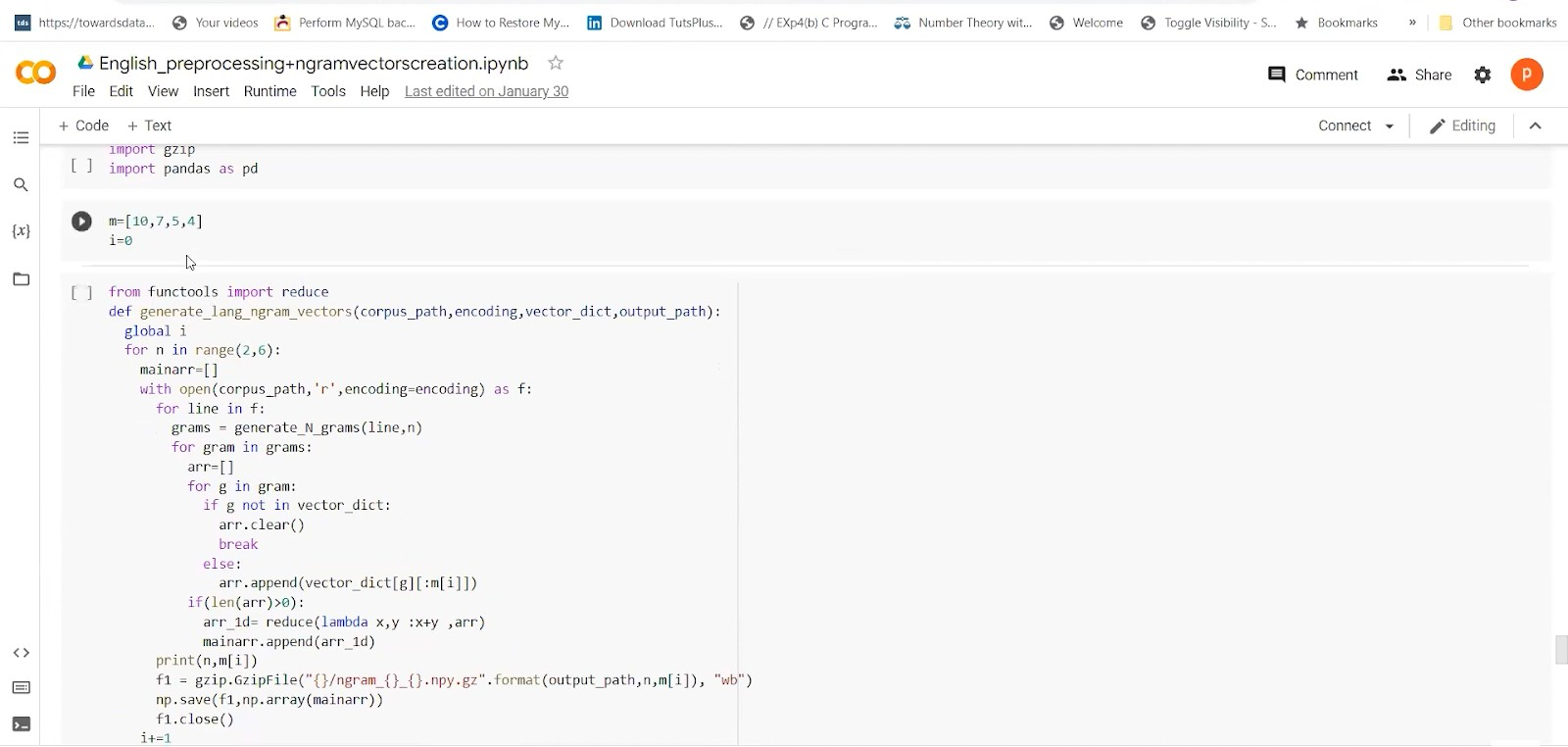Below is the pre-processing and creation of word vectors for English. We will use a similar process that can be followed for Hindi also.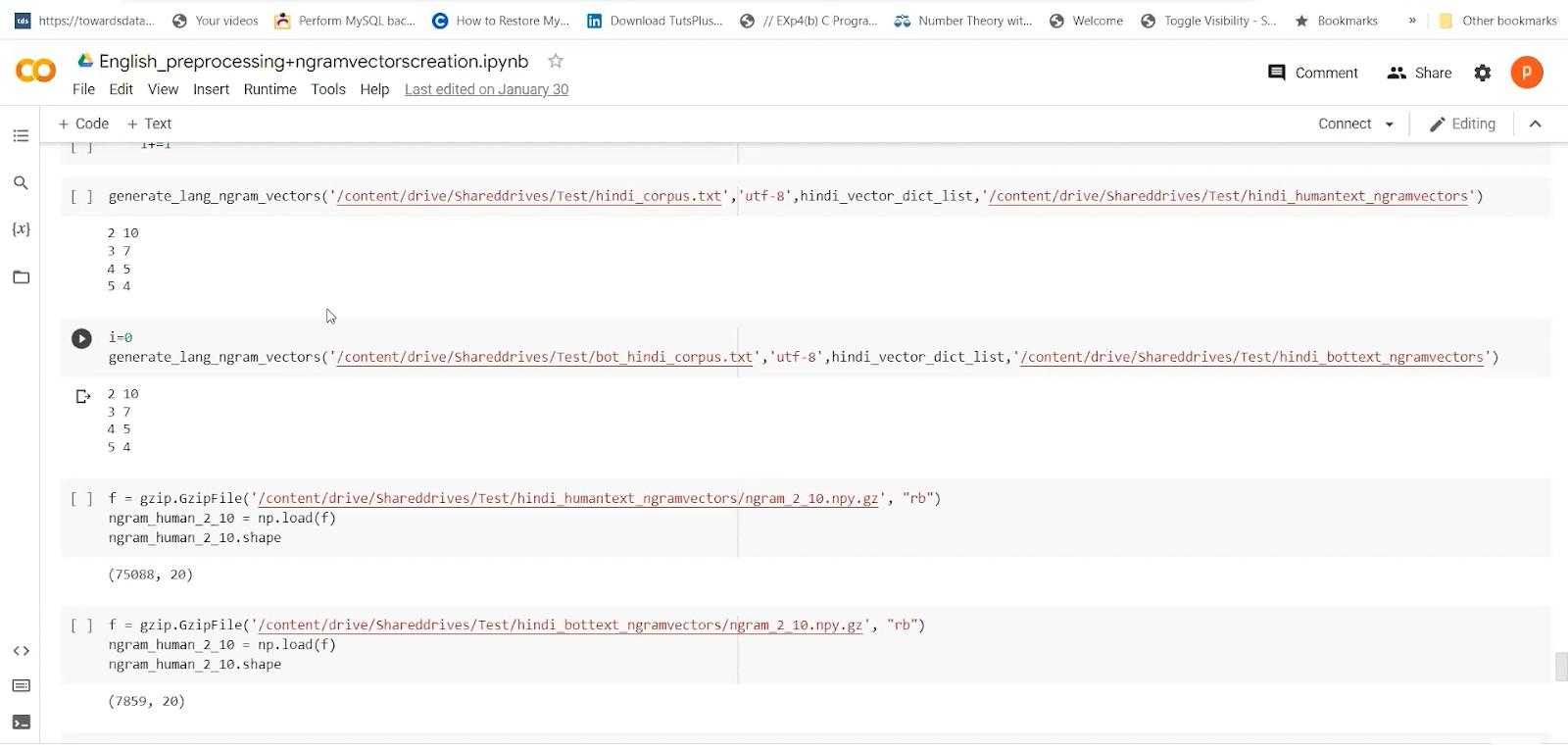## Bot Text Generation

Coming to the bot text generation, we first have to create the English dictionary from the corpus. We will get the characters to index arrays. It will help in getting the list of unique characters. Now set the SEQ_LEN as 256 and BATCH_SIZE as 16. We have a Prediction length to be 200 by default. Temperature parameters (temp. Is 0.3) will control the randomness of prediction by our models. If the temperature is lower, then the prediction will be less random and more accurate.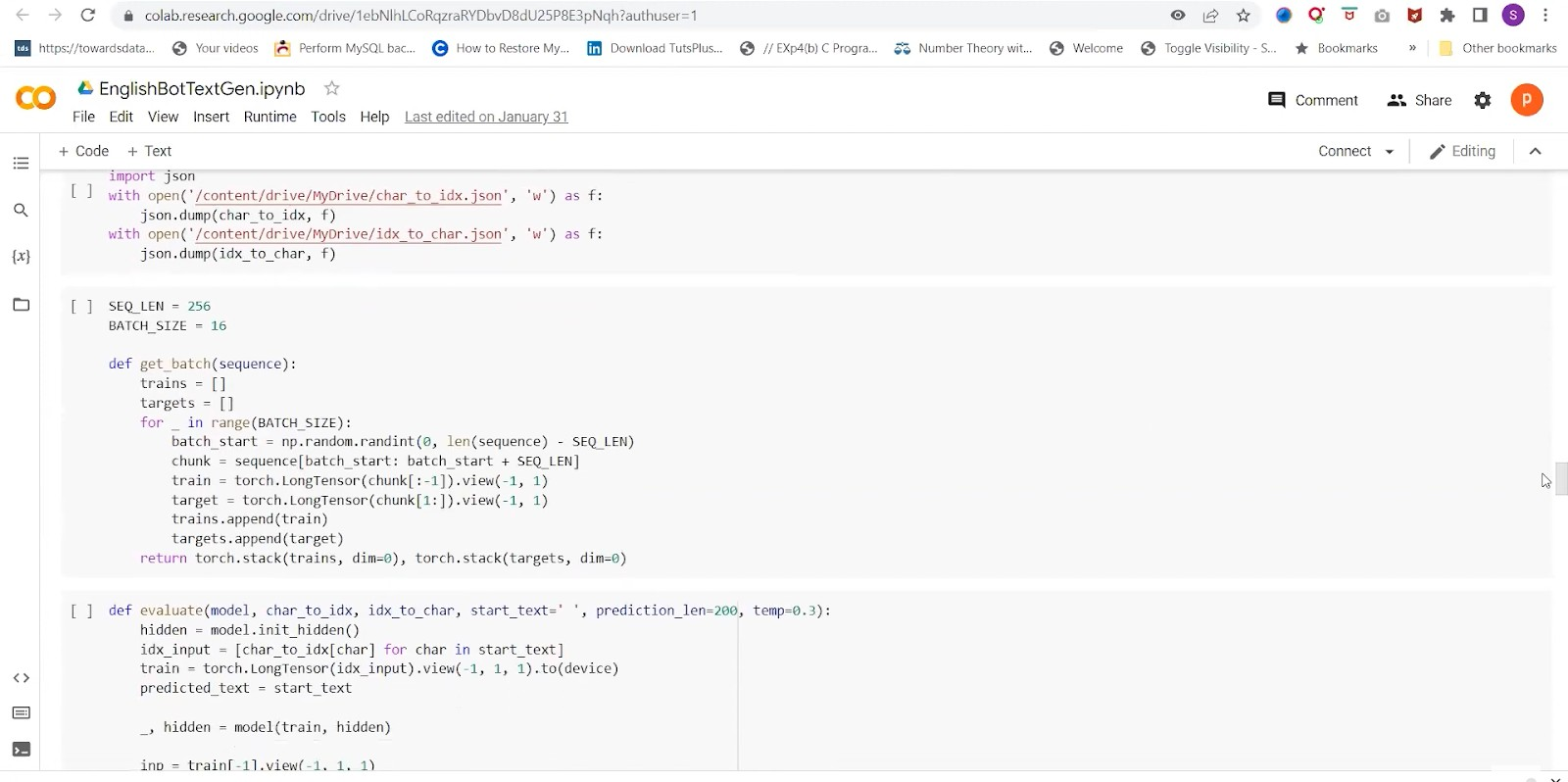Additionally, Layers would come into action in the forward method as we have to do some squeeze and un-squeeze because we have to compress some dimensions and elongate them based on the input sequence. Lastly, we will initialize the hidden layer, and it will be vector 0 0. The Dimensions will be hidden. We are moving it to devices like CPU and GPU. We are using torch.device and checking if we have a GPU available or not. Initialize the character level RNN with required hyperparameters. We have selected the first line from the human corpus, which is the input sequence for getting the predicted sequence from our character level.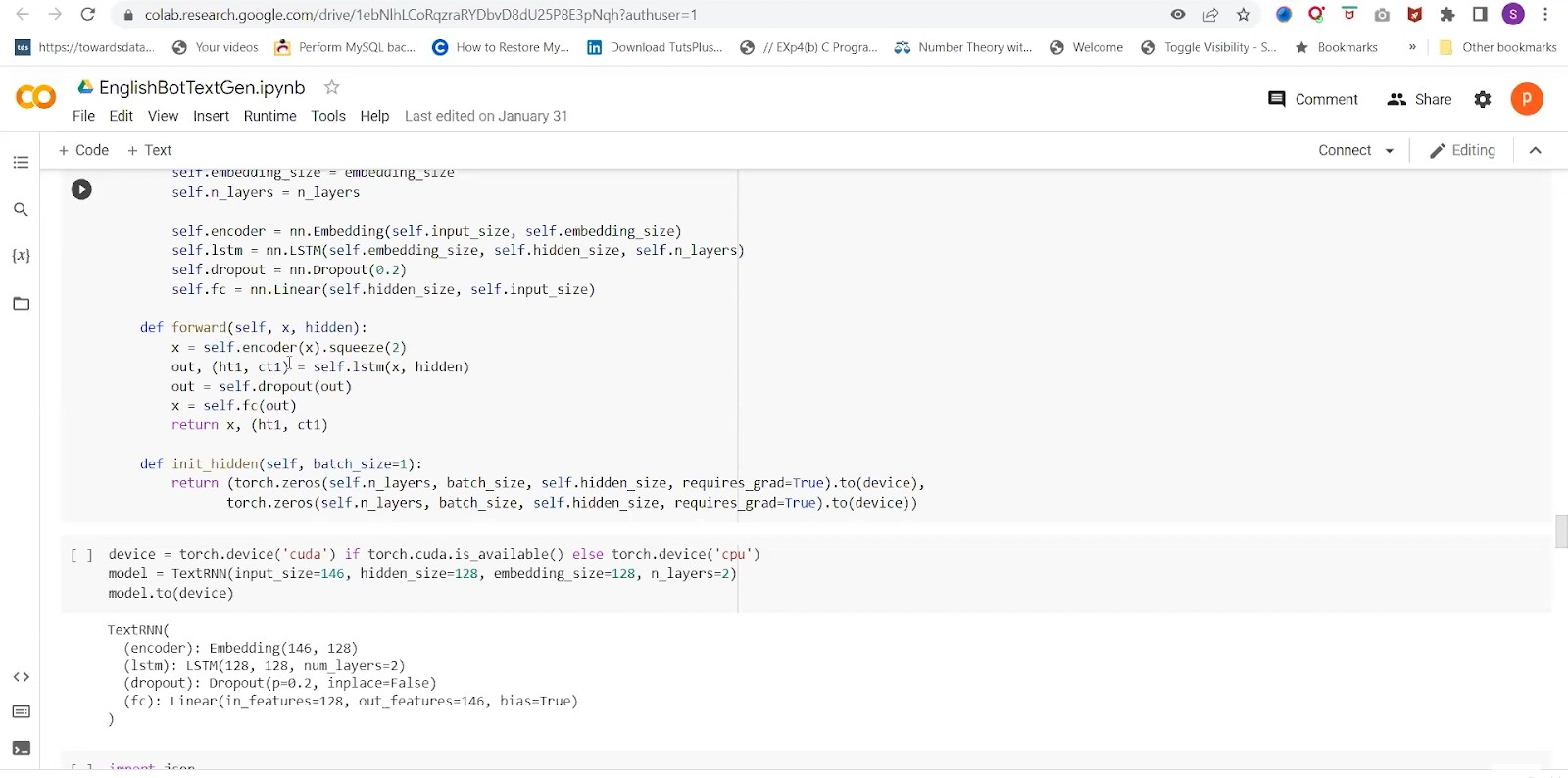We would create the training procedure by initializing our criteria. Also, it is a cross-entropy loss. We are passing the vector of indexes of characters that can be treated as the label encoding. The number of epochs is chosen as 10,000. We will train the model and then get the predicted output. Then, we can calculate the loss and backward Gradient using it. Set it up to Zero Gradient for evaluation.

Also, after the model has been trained, we can pass it on to the Evaluate function. We will use both characters to index the dictionary and index to character dictionary. The start text will be the first line of the English corpus or any random line. The prediction length can also be set up to 200 by default. The same pipeline could be used for Hindi also, but encoding would be utf-8. So after generating the bot files, we have to pass it to pre-processing steps and generation of n-gram vectors.

Now coming for the next step of Clustering those word vectors. We can use k-means or any density-based clustering method.

Now, we would set up the rapid cuda libraries. For hyperparameter tuning, we’re using tuna. It’s a type of basin optimization technique where we can use a TP sampler, grid samplers, and random samplers. We are proceeding with base n1 because it is faster. The cluster selection method for hdb scan in Cuda currently supports eom excess of mass. We are performing hbd scan on the best hyperparameters obtained above. Then we did the clustering procedure and got word vectors that are present in this cluster. Once we get the bot text for the human and bot corpus, we will compute some cluster matrix. However, one of the matrix will be computing the average distance in a cluster and dividing it by the number of combinations.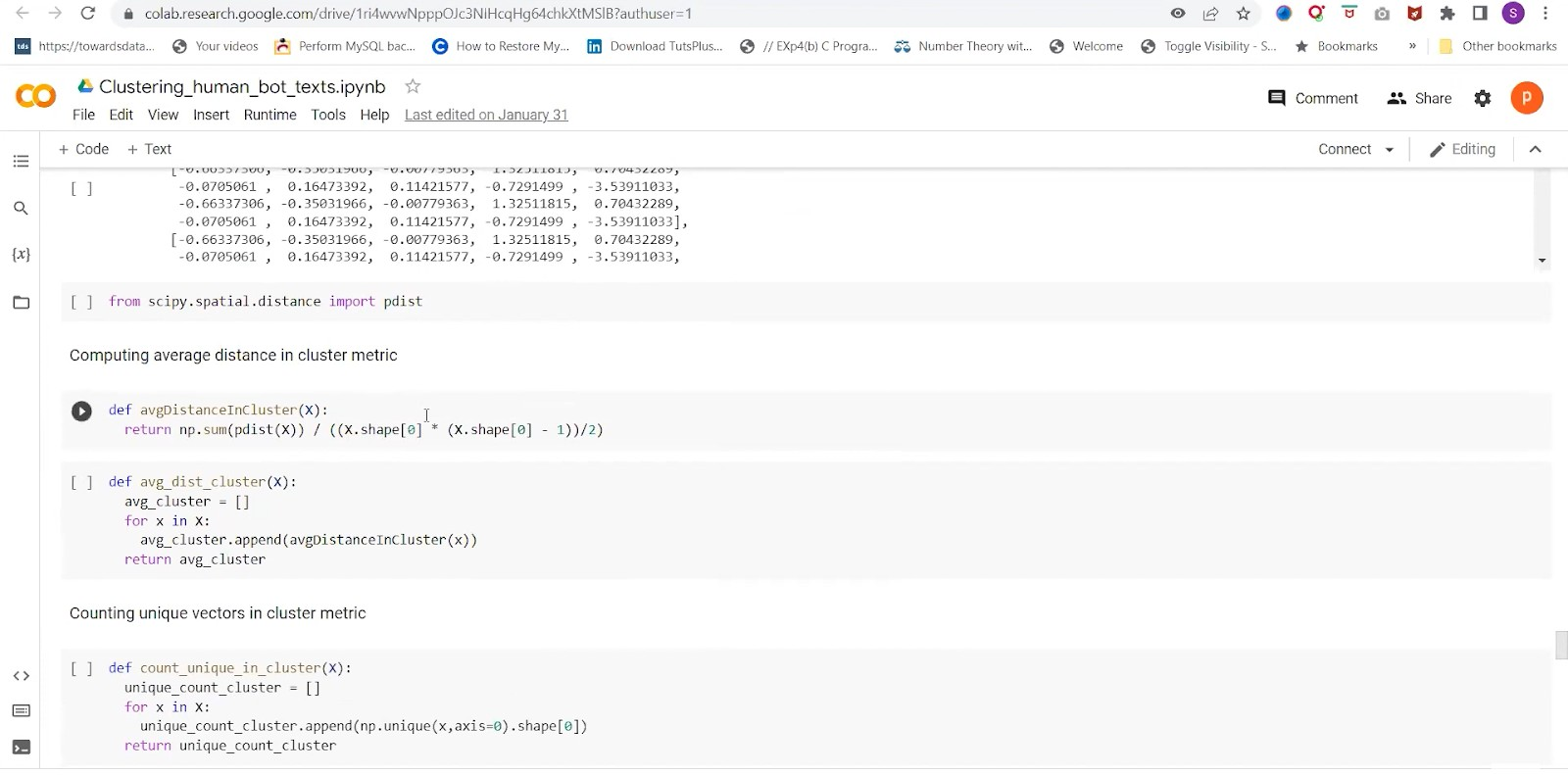We are using p-distance, the optimized computation pairwise for a given matrix. Now append those average distances of the clusters, and another metric would be counting the unique vectors. Let’s store all of this in a list for the human and bot corpus. Our null hypothesis is that if human and bot text corpuses are coming from the same population or not, an alternative is that they belong to different populations. We are selecting significant level alpha=0.05 and running the statistical test for 2 cluster metric lists which we obtained. By computing the p-value, if p is less than the significant level, our results will be statistically significant. We can reject the null hypothesis in favor of an alternate hypothesis. Before performing these steps, we will remove the noise labels.

## Conclusion

• We observed that basically p-value of the test is less than or equal to 0.05. Thus, the null hypothesis should favor the alternative hypothesis.
• Additionally, our results are statistically significant.
• Our Experiment has sufficient evidence to support the two cluster metric distributions derived from different populations.